### Home > A2C > Chapter 12 > Lesson 12.3.1 > Problem12-125

12-125.
1. Give the exact value(s) for each of the unknowns (0 θ < 2π) in the equations below. Homework Help ✎

1.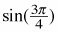= ?

2.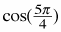=?

3. tanθ = 1

4. tan(θ) = −1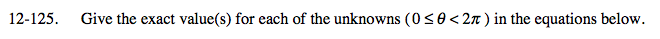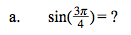Use the unit circle.

$\frac{\sqrt{2}}{2}$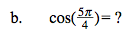See part (a).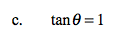$\text{Remember tan}\theta=\frac{\text{sin}\theta}{\text{cos}\theta}$

Where on the unit circle does sinθ = cosθ?

$\theta=\frac{\pi}{4},\,\frac{5\pi}{4}$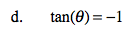See part (c).